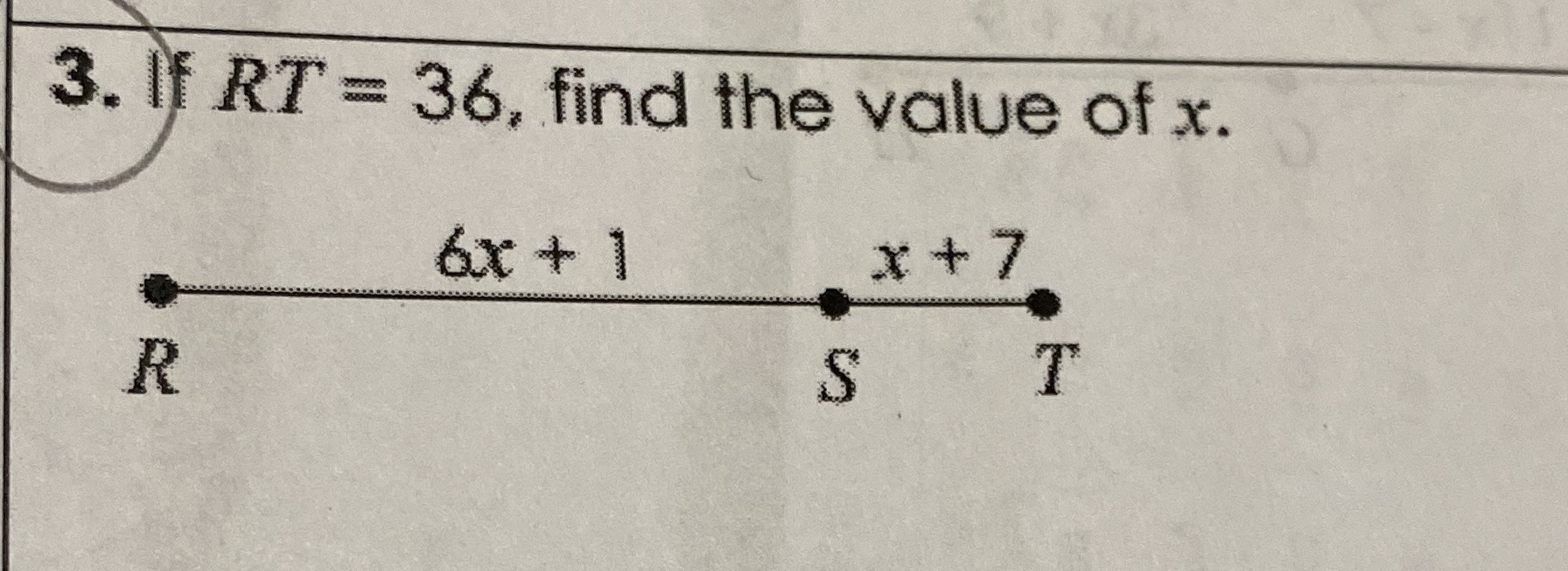Still have math questions?

If $$\mathrm{RT}=36$$, find the value of $$x$$.$$x=4$$